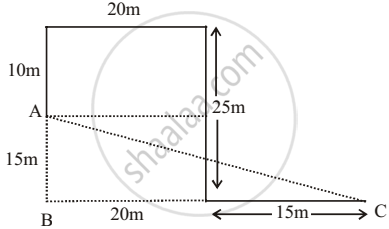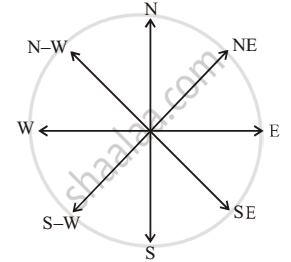# Pratham Travels 10 Metres in North Direction, He Turns Right and Travels 20 Metres. Again, He Turns Towards Right and Travels 25 Metres. Then, He Turns Towards Left and Travels 15 Metres. - Logical Reasoning

MCQ

Pratham travels 10 metres in North direction, he turns right and travels 20 metres. Again, he turns towards right and travels 25 metres. Then, he turns towards left and travels 15 metres. In which direction and how far is Pratham from his original position?

#### Options

• 35 metres, East

• 38.07 metres, South-East

• 25.08 metres, West

• 25 metres, North-East

#### Solution

38.07 metres, South-East

Explanation:A = starting point of Pratham

C = end point of pratham

Distance between AC = sqrt ("AB"^2 + "BC"^2)

=sqrt((15)^2 + (35)^2)

= sqrt(225+1225) = sqrt 1450 = 38.07 m

Therefore pratham is 38.07 m far from his original position and point C, which is end point is south- east from hos starting point A.

Concept: Direction and Distance Test (Entrance Exam)
Is there an error in this question or solution?